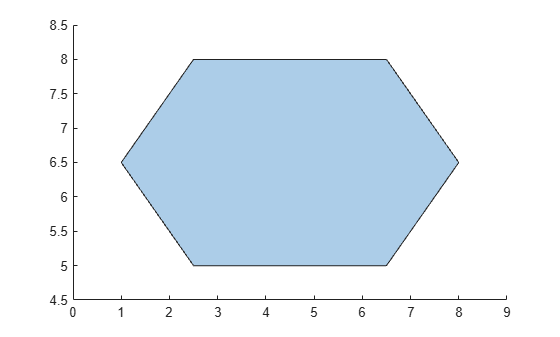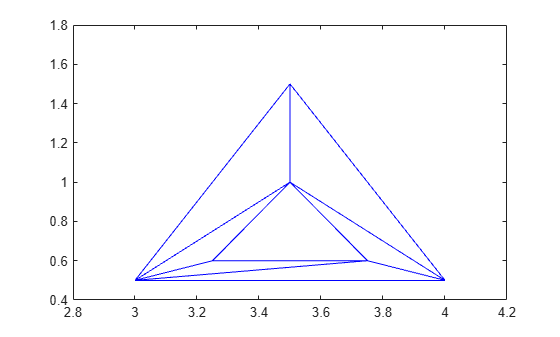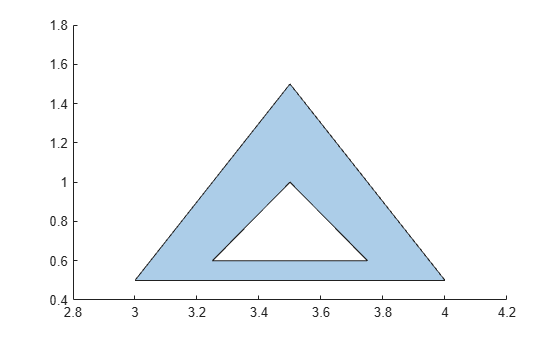# boundaryshape

Create `polyshape` from 2-D triangulation

Since R2018b

## Syntax

``polyout = boundaryshape(TR)``
``[polyout,vertexID] = boundaryshape(TR)``

## Description

example

````polyout = boundaryshape(TR)` creates a `polyshape` object from the boundary of a 2-D triangulation. `TR` can be either a `triangulation` object or a `delaunayTriangulation` object.Once you create the `polyshape` object `polyout`, you can analyze its properties or perform additional computations using `polyshape` functions. For example, you can access the vertices that define the boundary with the property `polyout.Vertices`, and you can plot the shape using the command `plot(polyout)`.```
````[polyout,vertexID] = boundaryshape(TR)` also returns a vector `vertexID` that maps the vertices of the `polyshape` to the vertices of the triangulation.```

## Examples

collapse all

Create and plot a simple `triangulation` object.

```P = [2.5 8.0; 6.5 8.0; 2.5 5.0; 6.5 5.0; 1.0 6.5; 8.0 6.5]; T = [5 3 1; 3 2 1; 3 4 2; 4 6 2]; TR = triangulation(T,P); triplot(TR) xlim([0 9]) ylim([4.5 8.5])```Create and plot a `polyshape` object whose boundary is equal to the boundary of the triangulation.

```polyout = boundaryshape(TR); plot(polyout)```Create and plot a `triangulation` object.

```T = [5 1 3; 6 1 4; 5 4 1; 2 6 3; 3 6 5; 2 1 6]; P = [3 0.5; 3.5 1.5; 4 0.5; 3.25 0.6; 3.75 0.6; 3.5 1]; TR = triangulation(T,P); triplot(TR) xlim([2.8 4.2]) ylim([0.4 1.8])```Create and plot a `polyshape` object whose boundaries are equal to the boundaries of the triangulation. Due to the inner and outer closed boundaries of the triangulation, `polyout` contains both a hole boundary and a solid boundary.

`polyout = boundaryshape(TR)`
```polyout = polyshape with properties: Vertices: [7x2 double] NumRegions: 1 NumHoles: 1 ```
`plot(polyout)`## Input Arguments

collapse all

Triangulation, specified as a 2-D `triangulation` object or a 2-D `delaunayTriangulation` object.

## Output Arguments

collapse all

Output `polyshape`, returned as a `polyshape` object.

Vertex identification, returned as a vector of integers. Each element in `vertexID` corresponds to the row number of the triangulation vertex in the `Points` property. The length of `vertexID` is equal to the number of rows in the `Vertices` property of the `polyshape` object.

Data Types: `double`

## Version History

Introduced in R2018b